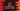# Java program to print the Neon numbers from 0 to 10000## Java program to print the Neon numbers from 0 to 10000:

In this post, I will show you how to print the Neon numbers from 0 to 10000 in Java. To solve this program, you need to know what is a Neon number and how to check if a number is Neon or not in Java.

Our program will have one function to check if a number is Neon or not. One loop will run from 0 to 10000 and it will use this function to check if the current iterating value is Neon or not. This loop will stop once the loop ends.

### Algorithm:

We will use the below algorithm:

• Run one loop from 0 to 10000.
• For each digit in the loop, check if it is a Neon number or not.
• If it is a Neon number, print its value.

### What is a Neon number:

A number is called a Neon number if the sum of digits of square of a number is equal to the number itself.

For example, 9 is a Neon number because square of 9 is 81 and the sum of digits of 81 is 8 + 1 = 9.

But, 11 is not a Neon number because square of 11 is 121 and the sum of digits of 121 is 4.

## Java program:

Below Java program prints the Neon numbers from 0 to 10000:

``````class Main {
static boolean isNeon(long n) {
long squareValue = n * n;
long sumOfDigits = 0;

while (squareValue > 0) {
sumOfDigits += squareValue % 10;
squareValue /= 10;
}

return sumOfDigits == n;
}

public static void main(String[] args) {
for (long i = 0; i <= 10000; i++) {
if (isNeon(i)) {
System.out.println(i);
}
}
}
}``````

### Explanation:

In this program,

• isNeon function is used to check if a number is Neon or not. This function takes one long value and returns one boolean. If the provided number is Neon, it returns true, else it returns false.

• squareValue variable holds the square of the number.
• sumOfDigits variable is to hold the sum of all digits of the number.
• The while loop is finding the sum of all digits. It gets the last digit of the number by using % and adds that to sumOfDigits. Then, it removes the last digit from the number.
• It returns true if sumOfDigits is equal to n. Else, it returns false.
• The for loop runs from 0 to 10000. For each number, it checks if that number is Neon or not. If it is a Neon number, it prints that.

If you run the above program, it will print the below output:

``````0
1
9``````

## Java program by using a while loop:

You can also write this program by using a while loop. It works in a similar way.

``````class Main {
static boolean isNeon(long n) {
long squareValue = n * n;
long sumOfDigits = 0;

while (squareValue > 0) {
sumOfDigits += squareValue % 10;
squareValue /= 10;
}

return sumOfDigits == n;
}

public static void main(String[] args) {
long i = 0;
while (i <= 10000) {
if (isNeon(i)) {
System.out.println(i);
}
i++;
}
}
}``````

We have replaced the for loop with a while loop in the main method. It runs until the value of i is less than or equal to 10000. For each value of i, it checks if the current value is Neon or not. If it is a Neon value, it prints that. The increment is done at the end of the while loop.

If you run this program, it will print the same output.

You can use any of these two methods we have discussed above. You can also try by changing the limit of the loop. But, it will always print 0, 1 and 9.